# Algebraic branch point

(diff) ← Older revision | Latest revision (diff) | Newer revision → (diff)

algebraic singular point

An isolated branch point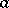of finite order of an analytic function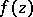, having the property that the limit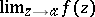exists for any regular element of continuation ofin a domain for whichis a boundary point. More exactly, a singular pointin the complex-plane for the complete analytic function, under continuation of some regular elementof this function with centre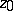along paths passing through, is called an algebraic branch point if it fulfills the following conditions: 1) There exists a positive numbersuch that the element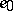may be extended along an arbitrary continuous curve lying in the annulus; 2) there exists a positive integer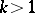such that if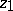is an arbitrary point of, the analytic continuation of the elementinyields exactlydifferent elements of the functionwith centre; ifis an arbitrary element with centre, all the remainingelements with centrecan be obtained by analytic continuation along closed paths around the point; and 3) the values at the pointsofof all elements which are obtainable fromby continuation intend to a definite, finite or infinite, limit astends towhile remaining in D.

The numberis said to be the order of the algebraic branch point. All branches of the functionobtainable by analytic continuation of the elementin the annulusmay be represented in a deleted neighbourhood ofby a generalized Laurent series (Puiseux series):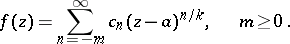The point at infinity,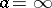, is called an algebraic branch point for a functionif the point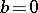is an algebraic branch point of the function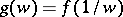.

There may exist several (and even an infinite number of) different algebraic branch points and regular points of a complete analytic function with a given affix.

How to Cite This Entry:
Algebraic branch point. Encyclopedia of Mathematics. URL: http://encyclopediaofmath.org/index.php?title=Algebraic_branch_point&oldid=14573
This article was adapted from an original article by E.D. Solomentsev (originator), which appeared in Encyclopedia of Mathematics - ISBN 1402006098. See original article